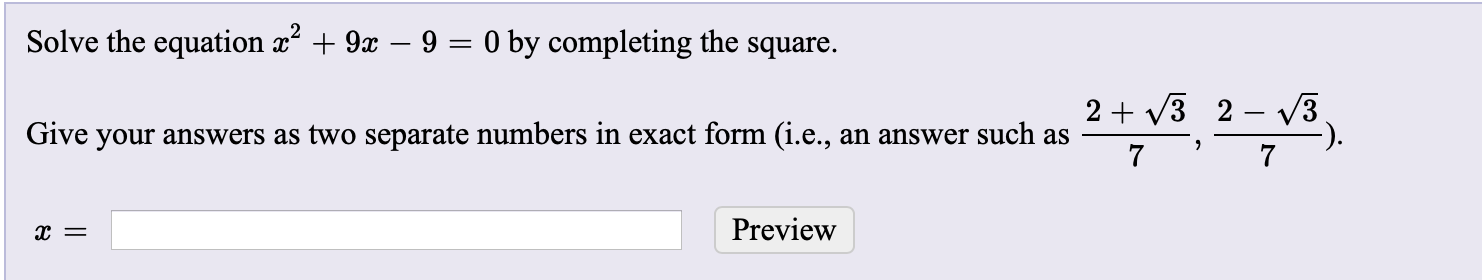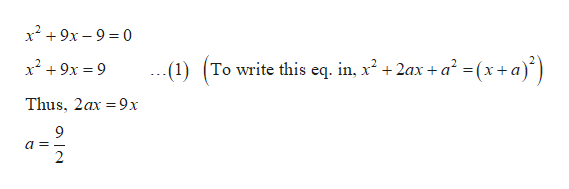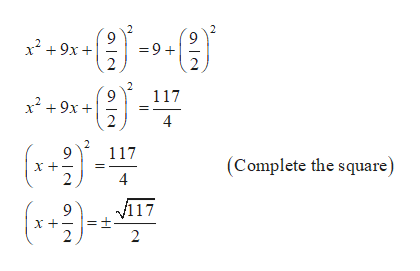# Solve the equation x + 9x – 9 = 0 by completing the square.2 + v3 2 – 3.Give your answers as two separate numbers in exact form (i.e., an answer such as7Previewх —

Question
27 viewshelp_outlineImage TranscriptioncloseSolve the equation x + 9x – 9 = 0 by completing the square. 2 + v3 2 – 3. Give your answers as two separate numbers in exact form (i.e., an answer such as 7 Preview х — fullscreen
check_circle

Step 1

The given equation is,

Step 2

Solve the equation by completing the square as follows.help_outlineImage Transcriptionclosex² + 9x – 9 = 0 -(1) (To write this eq. in, x² + 2ax + a² =(x+a)*) x² + 9x = 9 .. Thus, 2ax =9x %3D fullscreen
Step 3

Now, add the square of a to both th...help_outlineImage Transcriptionclosex + 9x + =9+ 117 x? +9x + 4 (**) (**)- 117 (Complete the square) 2 4 V117 x +- fullscreen

### Want to see the full answer?

See Solution

#### Want to see this answer and more?

Solutions are written by subject experts who are available 24/7. Questions are typically answered within 1 hour.*

See Solution
*Response times may vary by subject and question.
Tagged in

### Equations and In-equations